# Understanding Elementary Shapes (Exercise – 5.1)## Ex 5.1 Question 1.

What is the disadvantage in comparing line segments by mere observation?
Solution:

Comparing the line segment by mere observation may not be accurate.

## Ex 5.1 Question 2.

Why is it better to use a divider than a ruler, while measuring the length of a line segment?
Solution:

It is better to use a divider than a ruler while measuring the length of a line segment because if we use a ruler while measuring the length of a line segment we may have the following errors:

(i) The thickness of the ruler
(ii) Angular viewing

## Ex 5.1 Question 3.

Draw any line segment, say . Take any point C lying in between A and B. Measure the lengths of AB, BC and AC. Is AB = AC + CB?
[Note: If A, B, C are any three points on a line such AC + CB = AB, then we can be sure that C lies between A and B]
Solution:

AB is a line segment of length 7 cm

C is a point between A and B

Such that AC = 3 cm and CB = 4 cm.AC = 3 cm, CB = 4 cm.
∴ AC + CB = 3 cm + 4 cm = 7 cm.
But, AB = 7 cm.
So, AB = AC + CB.

## Ex 5.1 Question 4.

If A, B, C are three points on a line such that AB = 5 cm, BC = 3 cm and AC = 8 cm, which one of them lies between the other two?
Solution:

AB = 5 cm; BC = 3 cm
∴ AB + BC = 5 + 3 = 8 cm
But, AC = 8 cm
Hence, B lies between A and C.

## Ex 5.1 Question 5.

Verify, whether D is the mid point of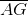.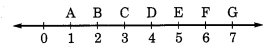Solution:

AG = 7 cm – 1 cm = 6 cm
AD = 4 cm – 1 cm = 3 cm
and DG = 7 cm – 4 cm = 3 cm
∴ AG = AD + DG.
Hence, D is the mid point of.

## Ex 5.1 Question 6.

If B is the mid point of  and C is the mid point of  , where A, B, C, D lie on a straight line, say why AB = CD?
Solution: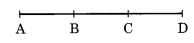B is the midpoint of$\overline { AC }$.
∴ AB = BC …..…(i)
C is the mid-point of$\overline { BD }$.
BC = CD ………..(ii)
Therefore from Eq.(i) and (ii)
AB = CD

## Ex 5.1 Question 7.

Draw five triangles and measure their sides. Check in each case, if the sum of the length of any two sides is always less than the third side.
Solution:

Case I. In ∆ABCAB = 2.5 cm
BC = 4.8 cm
and AC = 5.2 cm
AB + BC = 2.5 cm + 4.8 cm
= 7.3 cm
Since, 7.3 > 5.2
So, AB + BC > AC
Hence, sum of any two sides of a triangle is greater than the third side.

Case II. In ∆PQR,PQ = 2 cm
QR = 2.5 cm
and PR = 3.5 cm
PQ + QR = 2 cm + 2.5 cm = 4.5 cm
Since, 4.5 > 3.5
So, PQ + QR > PR
Hence, sum of any two sides of a triangle is greater than the third side.

Case III. In ∆XYZ,XY = 5 cm
YZ = 3 cm
and ZX = 6.8 cm
XY + YZ = 5 cm + 3 cm
= 8 cm
Since, 8 > 6.8
So, XY + YZ > ZX
Hence, the sum of any two sides of a triangle is greater than the third side.

Case IV. In ∆MNS,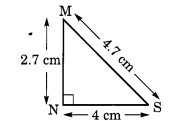MN = 2.7 cm
NS = 4 cm
MS = 4.7 cm
and MN + NS = 2.7 cm + 4 cm = 6.7 cm
Since, 6.7 >4.7
So, MN + NS > MS
Hence, the sum of any two sides of a triangle is greater than the third side.

Case V. In ∆KLM,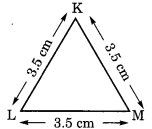KL = 3.5 cm
LM = 3.5 cm
KM = 3.5 cm
and KL + LM = 3.5 cm + 3.5 cm = 7 cm
7 cm > 3.5 cm
Solution:
(i) For one-fourth revolution, we have
So, KL + LM > KM
Hence, the sum of any two sides of a triangle is greater than the third side.
Hence, we conclude that the sum of any two sides of a triangle is never less than the third side.

error: Content is protected !!